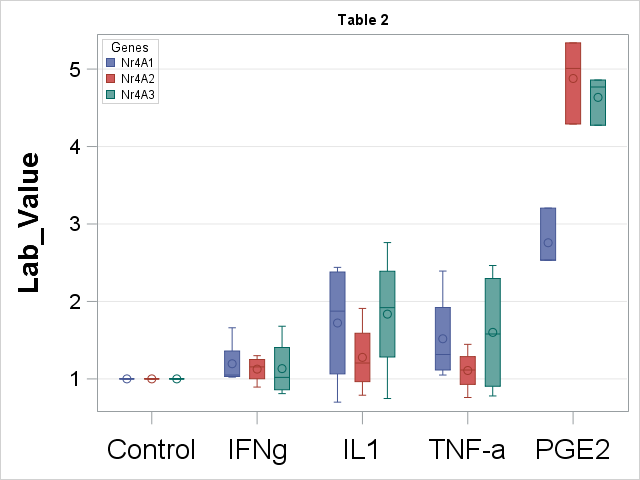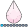Hi All,

I am using proc sgplot to plot a boxplot. but how can I add a star sign above the significance variables?

this is my boxplot figure, I want to add a sign in the last two box (red and green) to mark them as significant, since there P-value less than 0.05.Here is my code:

proc sgplot data=boxplot_1;
vbox lab_value / category = treatment group = group
lineattrs=(pattern=solid) whiskerattrs=(pattern=solid);
refline 0 / axis = y lineattrs = (color = black thickness = 2);
xaxis display=(nolabel) type = discrete labelattrs = (size = 15pt Weight = Bold) valueattrs = (size = 20pt);
yaxis grid labelattrs = (size = 20pt Weight = Bold) valueattrs = (size = 15pt);
keylegend / location = inside position = topleft across = 1;
run;

Any way to help me.

Thanks,

C

2 REPLIES 2art297
Opal | Level 21

Would using the annotate feature suffice? see: https://support.sas.com/resources/papers/proceedings11/277-2011.pdf

Art, CEO, AnalystFinder.comdjrisks
Barite | Level 11

Hello C,

Here is an example that you can use and edit. Basically you need to create the observations in your dataset at the correct y value and use the markerchar option:

``````data cars;
set sashelp.cars;
run;

data cars2;
invoicey = 100000; make = "Acura"; char = "*"; output;
invoicey = 100000; make = "Audi"; char = "*"; output;
invoicey = 100000; make = "BMW"; char = "*"; output;
run;

data cars3;
set cars cars2;
run;

proc sgplot data = cars3;
where make in ("Acura" "Audi" "BMW");
scatter x = make y = invoicey / markerchar = char markercharattrs=(size = 15 color = red);
vbox invoice / category = make;
run;``````

Many thanks,

Kriss

Discussion stats
• 2 replies
• 2178 views
• 0 likes
• 3 in conversation